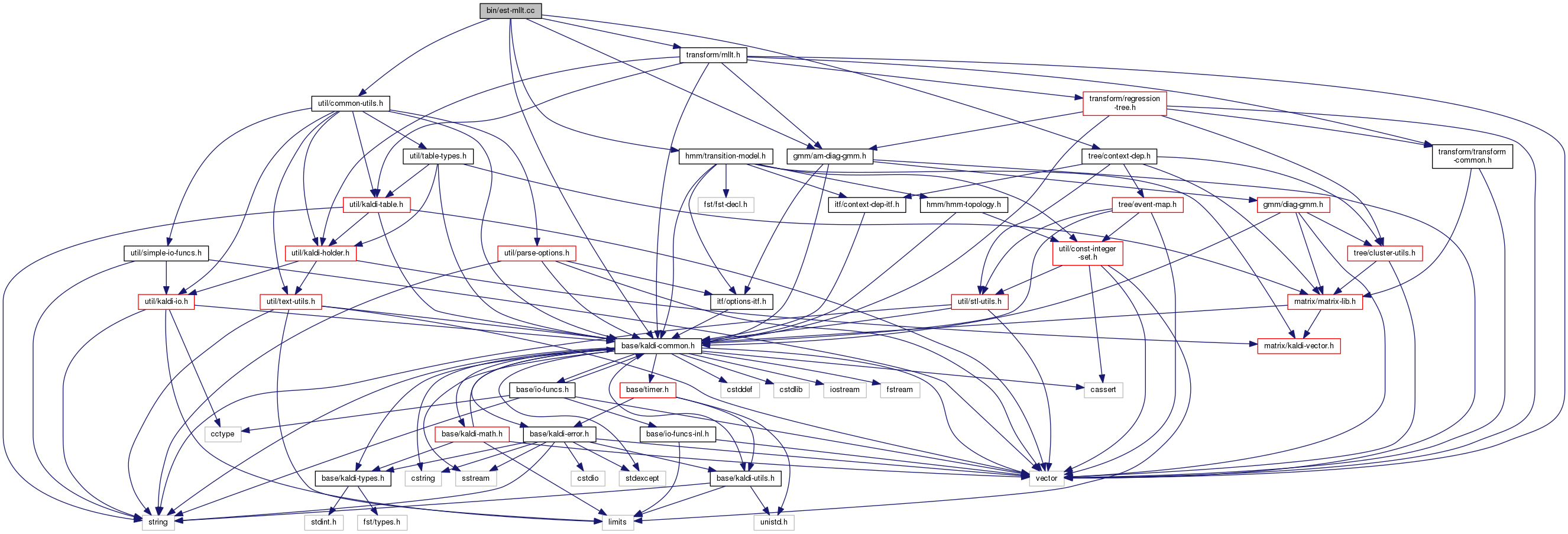est-mllt.cc File Reference
`#include "base/kaldi-common.h"`
`#include "util/common-utils.h"`
`#include "gmm/am-diag-gmm.h"`
`#include "tree/context-dep.h"`
`#include "hmm/transition-model.h"`
`#include "transform/mllt.h"`
Include dependency graph for est-mllt.cc:Go to the source code of this file.

## Functions

int main (int argc, char *argv[])

## ◆ main()

 int main ( int argc, char * argv[] )

Definition at line 26 of file est-mllt.cc.

26  {
27  try {
28  using namespace kaldi;
29  typedef kaldi::int32 int32;
30
31  const char *usage =
32  "Do update for MLLT (also known as STC)\n"
33  "Usage: est-mllt [options] <mllt-mat-out> <stats-in1> <stats-in2> ... \n"
34  "e.g.: est-mllt 2.mat 1a.macc 1b.macc ... \n"
35  "where the stats are obtained from gmm-acc-mllt\n"
36  "Note: use compose-transforms <mllt-mat-out> <prev-mllt-mat> to combine with previous\n"
37  " MLLT or LDA transform, if any, and\n"
38  " gmm-transform-means to apply <mllt-mat-out> to GMM means.\n";
39
40  bool binary = true; // write in binary if true.
41
42  ParseOptions po(usage);
43  po.Register("binary", &binary, "Write output in binary mode");
44
46
47  if (po.NumArgs() < 2) {
48  po.PrintUsage();
49  exit(1);
50  }
51
52  std::string mllt_out_filename = po.GetArg(1);
53
54  MlltAccs mllt_accs;
55  for (int32 i = 2; i <= po.NumArgs(); i++) {
56  std::string acc_filename = po.GetArg(i);
57  bool binary_in, add = true;
58  Input ki(acc_filename, &binary_in);
60  }
61
62  Matrix<BaseFloat> mat(mllt_accs.Dim(), mllt_accs.Dim());
63  mat.SetUnit();
64  BaseFloat objf_impr, count;
65  mllt_accs.Update(&mat, &objf_impr, &count);
66
67  KALDI_LOG << "Overall objective function improvement for MLLT is "
68  << (objf_impr/count) << " over " << count << " frames, logdet is "
69  << mat.LogDet();
70
71  Output ko(mllt_out_filename, binary);
72  mat.Write(ko.Stream(), binary);
73
74  return 0;
75  } catch(const std::exception &e) {
76  std::cerr << e.what() << '\n';
77  return -1;
78  }
79 }
This code computes Goodness of Pronunciation (GOP) and extracts phone-level pronunciation feature for...
Definition: chain.dox:20
Definition: mllt.cc:34
kaldi::int32 int32
void SetUnit()
Sets to zero, except ones along diagonal [for non-square matrices too].
void Update(MatrixBase< BaseFloat > *M, BaseFloat *objf_impr_out, BaseFloat *count_out) const
The Update function does the ML update; it requires that M has the right size.
Definition: mllt.h:69
const size_t count
float BaseFloat
Definition: kaldi-types.h:29
The class ParseOptions is for parsing command-line options; see Parsing command-line options for more...
Definition: parse-options.h:36
int32 Dim()
Definition: mllt.h:60
#define KALDI_LOG
Definition: kaldi-error.h:153
A class for estimating Maximum Likelihood Linear Transform, also known as global Semi-tied Covariance...
Definition: mllt.h:42